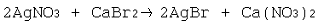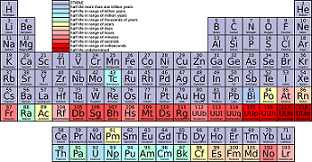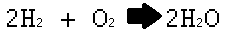# How to Interpret Chemical Equations

Instructor: Laura Foist

Laura has a Masters of Science in Food Science and Human Nutrition and has taught college Science.

What is a chemical equation and what we are able to determine from it? In this lesson, we will learn how the chemical equation tells us about quantity of atoms and molecules and about the structure of the molecule.

## Chemical Equations

In chemistry we will frequently see chemical equations like this one:These equations can be confusing at times. What do they really mean? What can we know about a reaction based on the chemical equation?

A chemical equation tells us what chemicals are needed to react together to form another chemical. In general, the things on the left side of the arrow are the reactants and the things on the right side of the arrow are the products.

## Chemical Equations and the Periodic Table

In order to understand chemical equations we need to understand a little bit about the periodic table.Each letter the periodic table is the abbreviation for a specific element. For example, H is for hydrogen, C is for carbon, and Ca is for calcium.

Not all abbreviations are as straight forward though For example, K is for Potassium which doesn't seem to make any sense since there isn't even a 'k' sound in potassium (this abbreviation actually comes from the Latin name for potassium, kalium). These abbreviations are used in chemical equations.

## Quantities of Each Chemical

Each chemical is made up of elements. The chemical formula can tell us how many atoms of each element are used. An atom is the simplest component of an element. Most of the time elements are found bulked together. We don't find a single element alone. Yet when speaking of a single element alone we refer to it as an atom.

So let's look at an example of making water.First we see that hydrogen (H) and oxygen (O) are used. Together they form dihydromonoxide, which is commonly referred to as water. 'Di-' means two, 'hydro' means hydrogens, 'mono' means one, and 'oxide' means oxygen. So a single water molecule has 2 hydrogen atoms and 1 oxygen atom. A molecule is the simplest component of a chemical. We don't typically find a single chemical molecule all by itself. But when we are referring to a single one we call it a molecule.

Notice the subscript '2' in the equation. If we have a jar full of hydrogen atoms, the hydrogen atoms don't float around by themselves. Two hydrogen atoms will combine together to form a hydrogen molecule. The same goes for oxygen.

There are some atoms (such as sodium) that won't combine with each other but hydrogen and oxygen will always combine together if they are left to float around together. This is why both hydrogen and oxygen have a little 2 next to them -- it denotes that we are using dihydrogen and dioxygen molecules.

We won't go into detail on how to balance an equation in this lesson, but just know that we have to end up with the same number of atoms on both sides of the arrow. You'll notice that we have two molecules of dihydrogen left of the arrow, and we end up with two molecules of water (H20). This is shown by having a large 2 before the dihydrogen molecule and a large 2 before the water molecule. When there is no number before the molecule then we know that this means that only 1 molecule was used. Since the dioxygen molecule has no number before it, we know that only 1 molecule of dioxygen was used.

Putting it all together: 2 molecules of hydrogen reacts with 1 molecule of oxygen to form 2 molecules of water.

## Another Example

Now let's look at a slightly more complex example:So starting at the beginning: the Ag refers to silver (yes another strange abbreviation that doesn't make sense based on the English word), the N is nitrogen, O is oxygen, Ca is calcium, and Br is bromide. To make naming easier we refer to the nitrogen and oxygen part (the nitro-trioxide) as nitrate. So the molecules that we have as reactants are: silver nitrate and calcium bromide. The molecules that are formed as products are: silver bromide and calcium nitrate.

Now we see that in the silver nitrate there is 1 atom of silver, 1 atom of nitrogen, and 3 atoms of oxygen. The calcium bromide has: 1 atom of calcium and 2 atoms of bromide. Then the silver bromide has: 1 atom of silver and 1 atom of bromide.

To unlock this lesson you must be a Study.com Member.

### Register to view this lesson

Are you a student or a teacher?

### Unlock Your Education

#### See for yourself why 30 million people use Study.com

##### Become a Study.com member and start learning now.
Back
What teachers are saying about Study.com

### Earning College Credit

Did you know… We have over 160 college courses that prepare you to earn credit by exam that is accepted by over 1,500 colleges and universities. You can test out of the first two years of college and save thousands off your degree. Anyone can earn credit-by-exam regardless of age or education level.